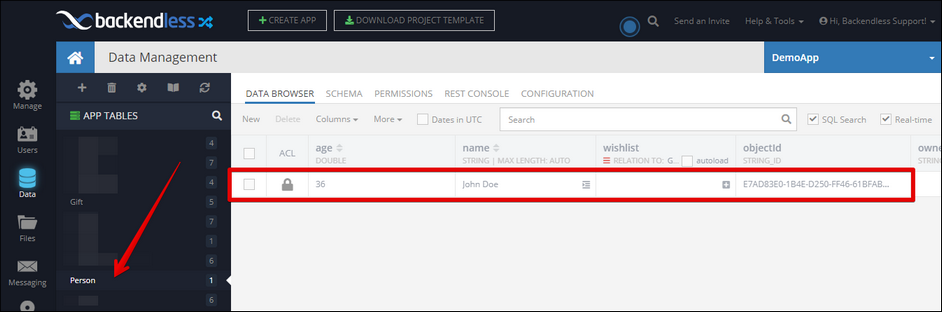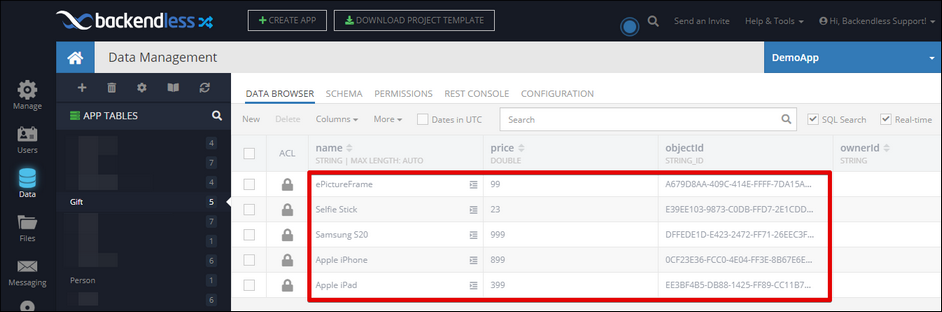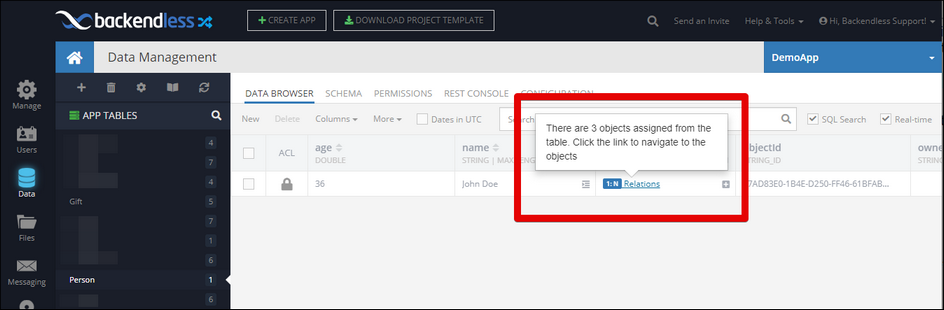# Setting a relation¶

This operation creates a relationship between a parent and child objects. If the parent object already has other related children, the relationship is reset - the child objects identified in this operation become the sole children of the parent. If you're looking for an operation which adds child objects to an existing set of children, see the Adding to a relation chapter of this guide.

Important

It is important that the relation column in the parent table is created before a transaction with the operation runs.

``````// Set the objects represented by the "children" argument
// as the children of the parent object.
// The "children" argument can be:
//   - a whereClause
//   - OpResult
//   - list of the objectId/Map/Custom class object values.
//
// The "parent" argument can be:
//   - a Map
//   - objectId
//   - custom class instance
//   - OpResult
//   - OpResultValueReference
unitOfWorkInstance.setRelation(dynamic parent,
String columnName,
dynamic children,
[String parentTable]);
``````

## Return Value¶

The operation returns an `OpResult` object which represents the result of this operation - number of child objects set in the relation.

## Examples¶

All examples below perform the same operation of linking objects from the `Gift` table to an object from the `Person` table through a column called `wishlist`. This is what the parent table looks like:You can see the `wishlist` column, which is a one-to-many relation with the `Gift` table shown below:The examples are organized by two levels: first is how the parent object is represented in the client program, then by the type of the child collection.

Parent object is represented as

The child objects are represented as

``````final unitOfWork = UnitOfWork();

// This is an array for child objects.
// They are referenced using objectId values.
// The example hard-codes the array, but in
// your code you'd get the objectId values
// from your data model or using a query
"EE3BF4B5-DB88-1425-FF89-CC11B7707500",
"0CF23E36-FCC0-4E04-FF3E-8B67E6E27200",
"DFFEDE1D-E423-2472-FF71-26EEC3F23700"];

// this is the objectId of the parent object.

// the name of the table where the parent object is stored
final parentTableName = "Person";

// the name of the relation column
final relationColumnName = "wishlist";

// add the setRelation call to the transaction
unitOfWork.setRelation(
personObjectId,
relationColumnName,
parentTableName
);

// run the transaction
unitOfWork.execute().then((result) => print("transaction complete - \$result"));
``````
``````final unitOfWork = UnitOfWork();

"objectId": "EE3BF4B5-DB88-1425-FF89-CC11B7707500",
};

final iPhone = {
"objectId": "0CF23E36-FCC0-4E04-FF3E-8B67E6E27200",
};

// This is a list of gift objects. These objects
// will be children in the relationship. As you
// can see the objects are constructed manually
// and their objectId's are set, however, in your
// application they would be loaded from the
// server. This is done here to keep the example
// more succinct.

// this is the objectId of the parent object.

// the name of the table where the parent object is stored
final parentTableName = "Person";

// the name of the relation column
final relationColumnName = "wishlist";

// add the setRelation call to the transaction
unitOfWork.setRelation(
personObjectId,
relationColumnName,
parentTableName
);

// run the transaction
unitOfWork.execute().then((result) => print("transaction complete - \$result"));
``````

``````final unitOfWork = UnitOfWork();

..objectId = "EE3BF4B5-DB88-1425-FF89-CC11B7707500";

..objectId = "0CF23E36-FCC0-4E04-FF3E-8B67E6E27200";

// This is a list of gift objects. These objects
// will be children in the relationship. As you
// can see the objects are constructed manually
// and their objectId's are set, however, in your
// application they would be loaded from the
// server. This is done here to keep the example
// more succinct.

// this is the objectId of the parent object.

// the name of the table where the parent object is stored
final parentTableName = "Person";

// the name of the relation column
final relationColumnName = "wishlist";

// add the setRelation call to the transaction
unitOfWork.setRelation(
personObjectId,
relationColumnName,
parentTableName
);

// run the transaction
unitOfWork.execute().then((result) => print("transaction complete - \$result"));
``````
The Gift class is shown below:
``````@reflector
String objectId;
String name;
double price;
}
``````

``````final unitOfWork = UnitOfWork();

// compose a query to fetch the gift objects
final queryBuilder = DataQueryBuilder();
queryBuilder.whereClause = "name in ( 'Apple iPad', 'Apple iPhone', 'Selfie Stick')";

// add the find operation to the transaction
// notice the result of the operation is saved in a variable -
// it will be used further in the setRelation operation

// this is the objectId of the parent object.

// the name of the table where the parent object is stored
final parentTableName = "Person";

// the name of the relation column
final relationColumnName = "wishlist";

// add the setRelation call to the transaction
unitOfWork.setRelation(
personObjectId,
relationColumnName,
parentTableName
);

// run the transaction
unitOfWork.execute().then((result) => print("transaction complete - \$result"));
``````

The child objects are represented as

``````final unitOfWork = UnitOfWork();

// This is an array for child objects.
// They are referenced using objectId values.
// The example hard-codes the array, but in
// your code you'd get the objectId values
// from your data model or using a query
"EE3BF4B5-DB88-1425-FF89-CC11B7707500",
"0CF23E36-FCC0-4E04-FF3E-8B67E6E27200",
"DFFEDE1D-E423-2472-FF71-26EEC3F23700"];

// this is the parent object. The only property that
// matters for the sake of establishing a relation
// is objectId. In your program you would be
// retrieving the object from the server. The
// technique shown here is for code brevity.
final personObject = {
};

// the name of the table where the parent object is stored
final parentTableName = "Person";

// the name of the relation column
final relationColumnName = "wishlist";

// add the setRelation call to the transaction
unitOfWork.setRelation(
personObject,
relationColumnName,
parentTableName
);

// run the transaction
unitOfWork.execute().then((result) => print("transaction complete - \$result"));
``````
``````final unitOfWork = UnitOfWork();

"objectId": "EE3BF4B5-DB88-1425-FF89-CC11B7707500"
};

final iPhone = {
"objectId": "0CF23E36-FCC0-4E04-FF3E-8B67E6E27200"
};

// This is a list of gift objects. These objects
// will be children in the relationship. As you
// can see the objects are constructed manually
// and their objectId's are set, however, in your
// application they would be loaded from the
// server. This is done here to keep the example
// more succinct.

// this is the parent object. The only property that
// matters for the sake of establishing a relation
// is objectId. In your program you would be
// retrieving the object from the server. The
// technique shown here is for code brevity.
final personObject = {
};

// the name of the table where the parent object is stored
final parentTableName = "Person";

// the name of the relation column
final relationColumnName = "wishlist";

// add the setRelation call to the transaction
unitOfWork.setRelation(
personObject,
relationColumnName,
parentTableName
);

// run the transaction
unitOfWork.execute().then((result) => print("transaction complete - \$result"));
``````

``````final unitOfWork = UnitOfWork();

..objectId = "EE3BF4B5-DB88-1425-FF89-CC11B7707500";

..objectId = "0CF23E36-FCC0-4E04-FF3E-8B67E6E27200";

// This is a list of gift objects. These objects
// will be children in the relationship. As you
// can see the objects are constructed manually
// and their objectId's are set, however, in your
// application they would be loaded from the
// server. This is done here to keep the example
// more succinct.

// this is the parent object. The only property that
// matters for the sake of establishing a relation
// is objectId. In your program you would be
// retrieving the object from the server. The
// technique shown here is for code brevity.
final personObject = {
};

// the name of the table where the parent object is stored
final parentTableName = "Person";

// the name of the relation column
final relationColumnName = "wishlist";

// add the setRelation call to the transaction
unitOfWork.setRelation(
personObject,
relationColumnName,
parentTableName);

// run the transaction
unitOfWork.execute().then((result) => print("transaction complete - \$result"));
``````
The Gift class is shown below:
``````@reflector
String objectId;
String name;
double price;
}
``````

``````final unitOfWork = UnitOfWork();

// compose a query to fetch the gift objects
final queryBuilder = DataQueryBuilder();
queryBuilder.whereClause = "name in ( 'Apple iPad', 'Apple iPhone', 'Selfie Stick')";

// add the find operation to the transaction
// notice the result of the operation is saved in a variable -
// it will be used further in the setRelation operation

// this is the parent object. The only property that
// matters for the sake of establishing a relation
// is objectId. In your program you would be
// retrieving the object from the server. The
// technique shown here is for code brevity.
final personObject = {
};

// the name of the table where the parent object is stored
final parentTableName = "Person";

// the name of the relation column
final relationColumnName = "wishlist";

// add the setRelation call to the transaction
unitOfWork.setRelation(
personObject,
relationColumnName,
parentTableName
);

// run the transaction
unitOfWork.execute().then((result) => print("transaction complete - \$result"));
``````

The child objects are represented as

``````final unitOfWork = UnitOfWork();

// This is an array for child objects.
// They are referenced using objectId values.
// The example hard-codes the array, but in
// your code you'd get the objectId values
// from your data model or using a query
"EE3BF4B5-DB88-1425-FF89-CC11B7707500",
"0CF23E36-FCC0-4E04-FF3E-8B67E6E27200",
"DFFEDE1D-E423-2472-FF71-26EEC3F23700"];

// this is the parent object. The only property that
// matters for the sake of establishing a relation
// is objectId. In your program you would be
// retrieving the object from the server. The
// technique shown here is for code brevity.
final personObject = Person()

// the name of the relation column
final relationColumnName = "wishlist";

// add the setRelation call to the transaction
unitOfWork.setRelation(
personObject,
relationColumnName,
);

// run the transaction
unitOfWork.execute().then((result) => print("transaction complete - \$result"));
``````
``````final unitOfWork = UnitOfWork();

"objectId": "EE3BF4B5-DB88-1425-FF89-CC11B7707500"
};

final iPhone = {
"objectId": "0CF23E36-FCC0-4E04-FF3E-8B67E6E27200"
};

// This is a list of gift objects. These objects
// will be children in the relationship. As you
// can see the objects are constructed manually
// and their objectId's are set, however, in your
// application they would be loaded from the
// server. This is done here to keep the example
// more succinct.

// this is the parent object. The only property that
// matters for the sake of establishing a relation
// is objectId. In your program you would be
// retrieving the object from the server. The
// technique shown here is for code brevity.
final personObject = Person()

// the name of the relation column
final relationColumnName = "wishlist";

// add the setRelation call to the transaction
unitOfWork.setRelation(
personObject,
relationColumnName,
);

// run the transaction
unitOfWork.execute().then((result) => print("transaction complete - \$result"));
``````

``````final unitOfWork = UnitOfWork();

..objectId = "EE3BF4B5-DB88-1425-FF89-CC11B7707500";

..objectId = "0CF23E36-FCC0-4E04-FF3E-8B67E6E27200";

// This is a list of gift objects. These objects
// will be children in the relationship. As you
// can see the objects are constructed manually
// and their objectId's are set, however, in your
// application they would be loaded from the
// server. This is done here to keep the example
// more succinct.

// this is the parent object. The only property that
// matters for the sake of establishing a relation
// is objectId. In your program you would be
// retrieving the object from the server. The
// technique shown here is for code brevity.
final personObject = Person()

// the name of the relation column
final relationColumnName = "wishlist";

// add the setRelation call to the transaction
unitOfWork.setRelation(
personObject,
relationColumnName,
);

// run the transaction
unitOfWork.execute().then((result) => print("transaction complete - \$result"));
``````
The Gift class is shown below:
``````@reflector
String objectId;
String name;
double price;
}
``````

``````final unitOfWork = UnitOfWork();

// compose a query to fetch the gift objects
final queryBuilder = DataQueryBuilder();
queryBuilder.whereClause = "name in ( 'Apple iPad', 'Apple iPhone', 'Selfie Stick')";
// add the find operation to the transaction
// notice the result of the operation is saved in a variable -
// it will be used further in the setRelation operation

// this is the parent object. The only property that
// matters for the sake of establishing a relation
// is objectId. In your program you would be
// retrieving the object from the server. The
// technique shown here is for code brevity.
final personObject = Person()

// the name of the relation column
final relationColumnName = "wishlist";

// add the setRelation call to the transaction
unitOfWork.setRelation(
personObject,
relationColumnName,
);

// run the transaction
unitOfWork.execute().then((result) => print("transaction complete - \$result"));
``````

The child objects are represented as

``````final unitOfWork = UnitOfWork();

// This is an array for child objects.
// They are referenced using objectId values.
// The example hard-codes the array, but in
// your code you'd get the objectId values
// from your data model or using a query
"EE3BF4B5-DB88-1425-FF89-CC11B7707500",
"0CF23E36-FCC0-4E04-FF3E-8B67E6E27200",
"DFFEDE1D-E423-2472-FF71-26EEC3F23700"];

// compose a query to retrieve the person
// from the database
final queryBuilder = DataQueryBuilder();

// this is the query which identifies the person of interest,
// this person object will be used as the parent in the relationship
queryBuilder.whereClause = "name = 'John Doe' and age = 36";

// add the object retriefinal operation to the transaction
final findResult = unitOfWork.find("Person", queryBuilder);

// since "findResult" references a collection of objects,
// get the first one from the collection. The reason we need to
// do this is because the setRelation operation below
// expects only one parent object. We know the first object
// will be the parent, that's why the "resolveTo" index is 0.
final paarentObjectRef = findResult.resolveTo(resultIndex: 0);

// the name of the relation column
final relationColumnName = "wishlist";

// add the setRelation call to the transaction
unitOfWork.setRelation(
paarentObjectRef,
relationColumnName,
);

// run the transaction
unitOfWork.execute().then((result) => print("transaction complete - \$result"));
``````
``````final unitOfWork = UnitOfWork();

"objectId": "EE3BF4B5-DB88-1425-FF89-CC11B7707500"
};

final iPhone = {
"objectId": "0CF23E36-FCC0-4E04-FF3E-8B67E6E27200"
};

// This is a list of gift objects. These objects
// will be children in the relationship. As you
// can see the objects are constructed manually
// and their objectId's are set, however, in your
// application they would be loaded from the
// server. This is done here to keep the example
// more succinct.

// compose a query to retrieve the person
// from the database
final queryBuilder = DataQueryBuilder();
// this is the query which identifies the person of interest,
// this person object will be used as the parent in the relationship
queryBuilder.whereClause = "name = 'John Doe' and age = 36";

// add the object retriefinal operation to the transaction
final findResult = unitOfWork.find("Person", queryBuilder);

// since "findResult" references a collection of objects,
// get the first one from the collection. The reason we need to
// do this is because the setRelation operation below
// expects only one parent object. We know the first object
// will be the parent, that's why the "resolveTo" index is 0.
final parentObjectRef = findResult.resolveTo(resultIndex: 0);

// the name of the relation column
final relationColumnName = "wishlist";

// add the setRelation call to the transaction
unitOfWork.setRelation(
parentObjectRef,
relationColumnName,

// run the transaction
unitOfWork.execute().then((result) => print("transaction complete - \$result"));
``````

``````final unitOfWork = UnitOfWork();
..objectId = "EE3BF4B5-DB88-1425-FF89-CC11B7707500";

..objectId = "0CF23E36-FCC0-4E04-FF3E-8B67E6E27200";

// This is a list of gift objects. These objects
// will be children in the relationship. As you
// can see the objects are constructed manually
// and their objectId's are set, however, in your
// application they would be loaded from the
// server. This is done here to keep the example
// more succinct.

// compose a query to retrieve the person
// from the database
final queryBuilder = DataQueryBuilder();
// this is the query which identifies the person of interest,
// this person object will be used as the parent in the relationship
queryBuilder.whereClause = "name = 'John Doe' and age = 36";

// add the object retriefinal operation to the transaction
final findResult = unitOfWork.find("Person", queryBuilder);

// since "findResult" references a collection of objects,
// get the first one from the collection. The reason we need to
// do this is because the setRelation operation below
// expects only one parent object. We know the first object
// will be the parent, that's why the "resolveTo" index is 0.
final parentObjectRef = findResult.resolveTo(resultIndex: 0);

// the name of the relation column
final relationColumnName = "wishlist";

// add the setRelation call to the transaction
unitOfWork.setRelation(
parentObjectRef,
relationColumnName,
);

// run the transaction
unitOfWork.execute().then((result) => print("transaction complete - \$result"));
``````
The Gift class is shown below:
``````@reflector
String objectId;
String name;
double price;
}
``````

``````final unitOfWork = UnitOfWork();

// compose a query to fetch the gift objects
final queryBuilder = DataQueryBuilder();
queryBuilder.whereClause = "name in ( 'Apple iPad', 'Apple iPhone', 'Selfie Stick')";

// add the find operation to the transaction
// notice the result of the operation is saved in a variable -
// it will be used further in the setRelation operation
// compose a query to retrieve the person
// from the database
final parentQueryBuilder = DataQueryBuilder();
// this is the query which identifies the person of interest,
// this person object will be used as the parent in the relationship
parentQueryBuilder.whereClause = "name = 'John Doe' and age = 36";

// add the object retrieval operation to the transaction
final findResult = unitOfWork.find("Person", parentQueryBuilder);

// since "findResult" references a collection of objects,
// get the first one from the collection. The reason we need to
// do this is because the setRelation operation below
// expects only one parent object. We know the first object
// will be the parent, that's why the "resolveTo" index is 0.
final parentObjectRef = findResult.resolveTo(resultIndex: 0);

// the name of the relation column
final relationColumnName = "wishlist";

// add the setRelation call to the transaction
unitOfWork.setRelation(
parentObjectRef,
relationColumnName,
The screenshot below is what the `Person` object will look like in Backendless Console. Notice the `wishlist` relation column now indicates there are related child objects: